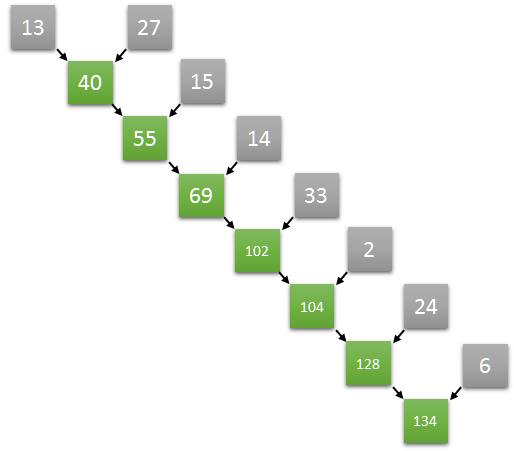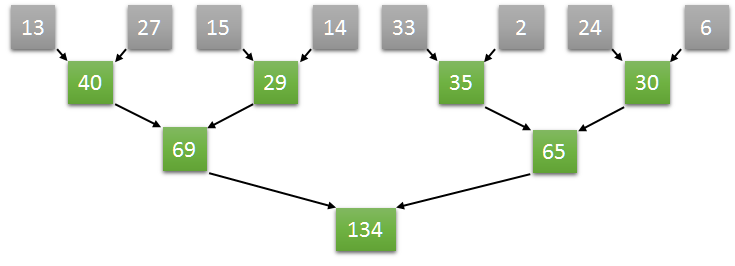Getting started with CUDA

# Implementing parallel reduction in CUDA

Reduction operations are those that reduce a collection of values to a single value. In this post, I will share how to implement parallel reduction operations using CUDA.

## Sequential Sum

Compute the sum of all elements of an array is an excellent example of the reduction operation. The sum of an array whose values are 13, 27, 15, 14, 33, 2, 24, and 6 is 134.

The interesting question is: How would you compute it? Probably your first answer would be doing something like this (((((((13+27)+15)+14)+33)+2)+24)+6). Am I right?The problem with this approach is that it is impossible to parallelize. Why? Each step depends on the result of the previous one.

## Parallel Sum

Adding values is an associative operation. So, we can try something like this ((13+27)+(15+14))+((33+2)+(24+6))This way is much better because now we can execute it in parallel!

## CUDA

Let’s figure out how to do it using CUDA.Here is the main idea:

• Assuming N as the number of the elements in an array, we start N/2 threads, one thread for every two elements
• Each thread computes the sum of the corresponding two elements, storing the result at the position of the first one.
• Iteratively, each step:
• the number of threads halved (for example, starting with 4, then 2, then 1)
• doubles the step size between the corresponding two elements (starting with 1, then 2, then 4)
• after some iterations, the reduction result will be stored in the first element of the array.

Let’s code

```#include "cuda_runtime.h"
#include "device_launch_parameters.h"

#include <iostream>
#include <numeric>
using namespace std;

__global__ void sum(int* input)
{

auto step_size = 1;

{
if (tid < number_of_threads) // still alive?
{
const auto fst = tid * step_size * 2;
const auto snd = fst + step_size;
input[fst] += input[snd];
}

step_size <<= 1;
}
}

int main()
{
const auto count = 8;
const int size = count * sizeof(int);
int h[] = {13, 27, 15, 14, 33, 2, 24, 6};

int* d;

cudaMalloc(&d, size);
cudaMemcpy(d, h, size, cudaMemcpyHostToDevice);

sum <<<1, count / 2 >>>(d);

int result;
cudaMemcpy(&result, d, sizeof(int), cudaMemcpyDeviceToHost);

cout << "Sum is " << result << endl;

getchar();

cudaFree(d);
delete[] h;

return 0;
}
```

## Time to action

In this post, we implemented a primary example of parallel reduction operation in CUDA. But, the pattern we adopted can be used in more sophisticated scenarios.

I strongly recommend you to try to implement other reduction operations (like discovering the max/min values of an array). Now, it would be easy. Right?

## Synchronizing Execution in Distributed Systems using RavenDB

1.##### Rolemberg

Boa noite.

Usando o conceito de renderfarm, digamos com 6 placas mães com processador…

Pego um arquivo de imagem para renderizar. Um arquivo de LUMION ou twinmontion, por exemplo…

Então eu leio e separo fragmentos desse arquivo pra renderizar em cada placa? É isso?

E depois como fazer para juntar esses fragmentos em uma imagem final?

2.##### Cheng Yu

There should be a __syncthreads() call at the end of the while loop of the kernel function.